# Pole (of a function)

(diff) ← Older revision | Latest revision (diff) | Newer revision → (diff)

An isolated singular pointof single-valued character of an analytic functionof the complex variablefor which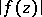increases without bound whenapproaches: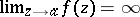. In a sufficiently small punctured neighbourhood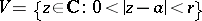of the point, orin the case of the point at infinity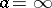, the functioncan be written as a Laurent series of special form: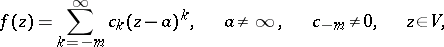(1)

or, respectively,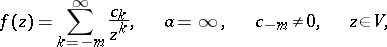(2)

with finitely many negative exponents if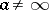, or, respectively, finitely many positive exponents if. The natural numberin these expressions is called the order, or multiplicity, of the pole; whenthe pole is called simple. The expressions (1) and (2) show that the functionif, or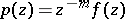if, can be analytically continued (cf. Analytic continuation) to a full neighbourhood of the pole, and, moreover,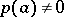. Alternatively, a poleof ordercan also be characterized by the fact that the function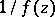has a zero of multiplicityat.

A pointof the complex space,, is called a pole of the analytic functionof several complex variablesif the following conditions are satisfied: 1)is holomorphic everywhere in some neighbourhoodofexcept at a set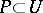,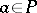; 2)cannot be analytically continued to any point of; and 3) there exists a function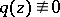, holomorphic in, such that the function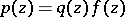, which is holomorphic in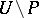, can be holomorphically continued to the full neighbourhood, and, moreover,. Here also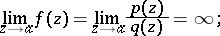however, for, poles, as with singular points in general, cannot be isolated.

How to Cite This Entry:
Pole (of a function). Encyclopedia of Mathematics. URL: http://encyclopediaofmath.org/index.php?title=Pole_(of_a_function)&oldid=15756
This article was adapted from an original article by E.D. Solomentsev (originator), which appeared in Encyclopedia of Mathematics - ISBN 1402006098. See original article